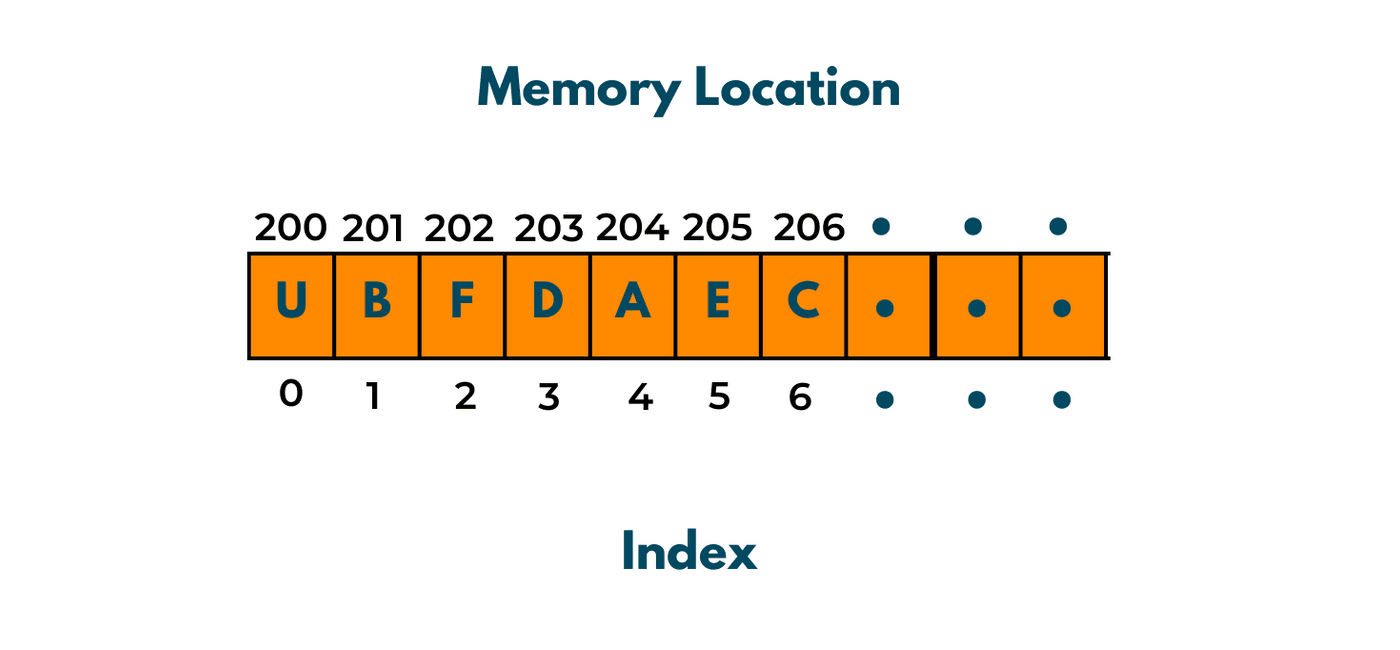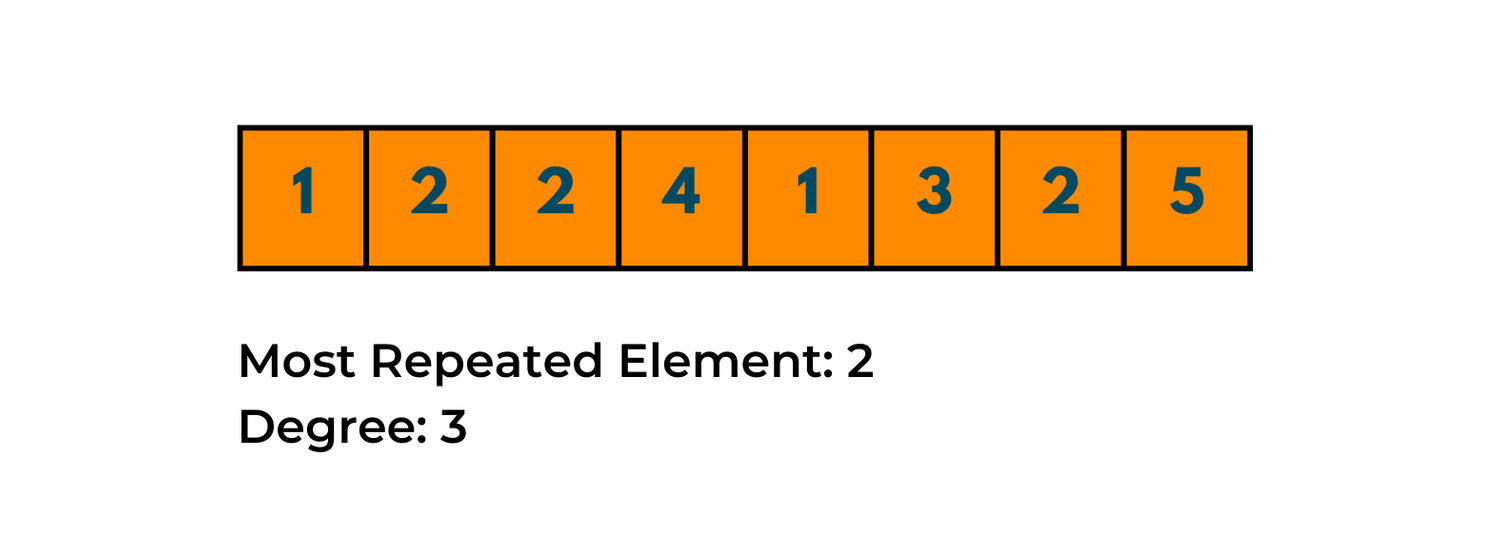# Degree of an Array Explained (Python & C++ code)

Probably the first data structure that you come across is arrays. We learned about arrays when we first start learning to code, and most of us have extensively used them in our codes. Today, we will talk about What is a degree of an Array and how to find it in Python and C++ with code.

## Degree of an Array

In technical jargon, arrays are a continuous list of elements that are of the same type. In simple words, consider ten elements that are all integers and are stored next to each other, these 10 elements form an integer array.

Arrays are used to store elements of the same type in continuous memory locations. This is done because it makes it easier to access the elements.A degree of an array is defined as the maximum number of repetitions of an element in the given array.

Too confusing? Let us have a look at an example. Let us consider an array, that has non-negative integers. The array is given as [1,2,2,4,1,3,2,5].

Now when you look at this array, what is the element that repeats the most? The element is "2". And if you are asked how many times does it repeat? Your answer will be 3. Therefore the degree of this array is 3. The degree for any array is the number of times a single item repeats.

### The Problem Statement

The degree of an array is a problem in which you are given a nonnegative integer array. Then you are asked to find the degree of the array and also the smallest subarray of the array that has the same degree as the array. Let us take an example to understand this problem.

Let us consider our previous array, [1,2,2,4,1,3,2,5]. Then the degree of this array is 3, and the most repeated element of this array is 2. Now we need to find the smallest subset of this array that has the same degree as the array.

In simpler words, we need to find the smallest subarray of this array in which "2" occurs the same number of times as in the main array. One point you must remember is that a subarray is different from a subset. In a subset, you can have elements in any order, but in a subarray, elements must be in the same order as in the main array.

For our example, [1,2,2,4,1,3,2,5],

The smallest subarray with the same degree is [2,2,4,1,3,2].## How to Find the Degree of an Array?

When you are given an array of finite numbers and told to find the degree of the array, what is the first task you should think about? The first task is to know how many times a given item occurs in the array. You also need to know the element that is repeated the most number of times.

After that, we need to find the subarray of the array that has the same degree as the main array. In order to do this, we need to revisit our example. We can maintain a dictionary of all the numbers that occur in the array and against the values the number of times that each number is repeated.

A dictionary in python is a collection of key: value pairs. In our case, the keys will be each unique number and the values will be the number of times each element is repeated in the array.

After we are done with this we will try to find the subarray that has the smallest length but the same degree as the array. In order to do this, we have two approaches, a brute force approach and an optimized approach.

### 01) Brute force Approach

In the brute force approach, we would find out all the possible subarrays of the given array. Then we would remove all the subarrays that do not have the same degree as the given array.

After we are done with this, we will find the length of all the subarrays that have the same degree as the original array. Then we would find the minimum value of all the lengths is provide that as output.

### 02) Optimised Approach

In the optimized approach, we will use intuition. If we look at our previous example, the subarray that had the same degree as the original array and the smallest length, was [2,2,4,1,3,2]. Do you observe a fact in it? The subarray begins with "2" and ends with "2".

That means the subarray that has the same degree as the original array and is the smallest in length will always start with the element that occurs the most number of times in the original array. Therefore when we go through the array to find its degree, we remember the left index of the element that has the highest number of repetitions.

Then when we find the element with the highest repetitions we will have its left-most index and its right-most index. We count the number of elements in between the two indexes and that gives us the length of the required sub-array.

### Python Implementation

The code for the optimized solution in Python 3:

```def smallsubarr(arr, n):

leftind = dict()
record = dict()
maxfreq,length, startind = 0, 0, 0

for i in range(n):
temp = arr[i]
if (temp not in record.keys()):
leftind[temp] = i
record[temp] = 1
else:
record[temp] += 1

if (record[temp] > maxfreq):
maxfreq = record[temp]
length = i - leftind[temp] + 1
startind = leftind[temp]

elif (record[temp] == maxfreq and
i - leftind[temp] + 1 < length):
length = i - leftind[temp] + 1
startind = leftind[temp]

result = len(arr[startind:startind+length])
print("The length of the smallest subarray is",result)

arr = []
n = int(input("Enter the length of the array: "))
print("Enter the elements of the array:")
for _ in range(n):
val = int(input())
arr.append(val)
smallsubarr(arr,n)
```

Output:

```Enter the length of the array: 5
Enter the elements of the array:
1
2
2
2
1
The length of the smallest subarray is 3
```

### C++ Implementation

The code for the optimized solution in C++:

```#include <bits/stdc++.h>
using namespace std;

void smallestSub(int a[], int n)
{
unordered_map<int, int> leftind;
unordered_map<int, int> counter;
int maxoccur = 0;
int mini, strind;
int length = 0;
for (int i = 0; i < n; i++) {

int x = a[i];
if (counter[x] == 0) {
leftind[x] = i;
counter[x] = 1;
}
else
counter[x]++;

if (counter[x] > maxoccur) {
maxoccur = counter[x];
mini = i - leftind[x] + 1;
strind = leftind[x];
}
else if (counter[x] == maxoccur && i - leftind[x] + 1 < mini) {
mini = i - leftind[x] + 1;
strind = leftind[x];
}
}
for (int i = strind; i < strind + mini; i++)
{
++length;
}
cout<<"The length of the smallest sub array is "<<length;

}

int main()
{
int n;
cout<<"Enter the number of elements\n";
cin>>n;
int arr[n];
cout<<"Enter the elements of the array\n";
for(int i=0;i<n;++i)
{
cin>>arr[i];
}
smallestSub(arr, n);
return 0;
}
```

Output:

```Enter the number of elements
5
Enter the elements of the array
1
2
2
2
1
The length of the smallest sub array is 3
```

## Conclusion

Arrays are used when we want to maintain a linear of elements in order. The degree of an array is the number of times the most repeated value occurs. Now you know how to find the degree of an array and the subarray that has the smallest length but the same degree as the original array using two methods: brute force and optimization method.

### FavTutor - 24x7 Live Coding Help from Expert Tutors!